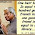# Vedic Maths Shortcut Methods for Multiplying with 9, 99, 999 and 9999 - Pdf Download

Friends, with this post we are giving you the pdf file of Vedic Mathematics Shortcut tricks. This file consists of very simple and fast techniques to multiply any number with 9, 99, 999 and 9999. These useful techniques of Vedic Mathematics are shared by our friend Hareesh Kumar. We thank him for his great efforts.  To be perfect in this technique one should be perfect in subtractions and even with the ‘9’ table.  We hope this will be useful for your upcoming competitive exams. All the Best :)

-->

1.12*9=12*(10-1)=12*10-12*1=120-12=108
43*99=43*(100-1)=43*100-43*1=4300-43=4257
236*999=236*(1000-1)=236*1000-236*1=236000-236=235764 etc

1.i think the both the methods are same...but the approach is different

2.Thank you friend! :)

3.thanks friend.

4.5.thank u so much...your contents are very much useful..

6.thank u so much.....your contents are very useful...